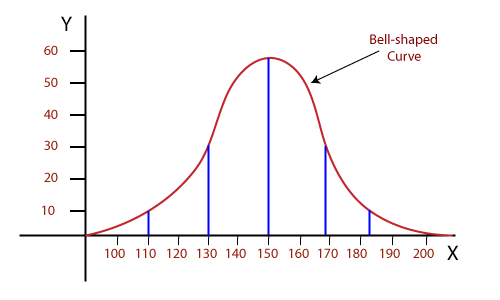# What do you understand by Normal distribution?

• If the given data is distributed around a central value in the bell-shaped curve without any left or right bias, then it is called Normal distribution. It is also called a Bell Curve because it looks like a bell?shaped curve.
• The normal distribution has a mean value, half of the data lies to the left of the curve, and half of the data lies right of the curve.
• In probability theory, the normal distribution is also called a Gaussian distribution, which is used for the probability distribution.
• It is a probability distribution function used to see the distribution of data over the given range.
• Normal distribution has two important parameters: mean(µ) and standard deviation(σ).